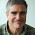## Tuesday, June 03, 2003

### Foundations of Complexity Lesson 19: The Immerman-Szelepcsenyi Theorem

Previous Lesson

In this lesson we will prove the Immerman-Szelepcsényi Theorem.

Theorem (Immerman-Szelepcsényi): For reasonable s(n)≥ log n, NSPACE(s(n))=co-NSPACE(s(n)).

Let M be a nondeterministic machine using s(n) space. We will create a nondeterministic machine N such that for all inputs x, N(x) accepts if and only if M(x) rejects.

Fix an input x and let s=s(|x|). The total number of configurations of M(x) can be at most cs for some constant c. Let t=cs. We can also bound the running time of M(x) by t because any computation path of length more than t must repeat a configuration and thus could be shortened.

Let I be the initial configuration of M(x). Let m be the number of possible configurations reachable from I on some nondeterministic path. Suppose we knew the value of m. We now show how N(x) can correctly determine that M(x) does not accept.

```Let r=0
For all nonaccepting configurations C of M(x)
Try to guess a computation path from I to C
If found let r=r+1
If r=m then accept o.w. reject
```
If M(x) accepts then there is some accepting configuration reachable from I so there must be less than m non-accepting configurations reachable from I so N(x) cannot accept. If M(x) rejects then there is no accepting configurations reachable from I so N(x) on some nondeterministic path will find all m nonaccepting paths and accept. The total space is at most O(s) since we are looking only at one configuration at a time.

Of course we cannot assume that we know m. To get m we use an idea called inductive counting. Let mi be the number of configurations reachable from I in at most i steps. We have m0=1 and mt=m. We show how to compute mi+1 from mi. Then starting at m0 we compute m1 then m2 all the way up to mt=m and then run the algorithm above.

Here is the algorithm to nondeterministically compute mi+1 from mi.

```Let mi+1=0
For all configurations C
Let b=0, r=0
For all configurations D
Guess a path from I to D in at most i steps
If found
Let r=r+1
If D=C or D goes to C in 1 step
Let b=1
If r<mi halt and reject
Let mi+1=mi+1+b
```
The test that r<mi guarantees that we have looked at all of the configurations D reachable from I in i steps. If we pass the test each time then we will have correctly computed b to be equal to 1 if C is reachable from I in at most i+1 steps and b equals 0 otherwise.

We are only remembering a constant number of configurations and variables so again the space is bounded by O(s). Since we only need to remember mi to get mi+1 we can run the whole algorithm in space O(s).

1.I am not sure I understand this algorithm. Why can't we just define the following non-deterministic turing machine N(x):

1. Non-deterministically guess the accepting configuration of M(x)
2. Verify the accepting configuration
3. If successful, then reject, otherwise accept

2.M(x) may have different nondeterministic choices, some lead to accept and others leading to reject. In this case both M(x) and N(x) will have (different) choices that lead to acceptance.

3.Let me try to modify the algorithm just a little:

Let r=0
For all accepting configurations C of M(x)
Try to guess a computation path from I to C
If found let r=r+1
If r>0 then reject o.w. accept

We are now iterating through all accepting configurations of M(x). The existence of a single reachable, accepting configuration is enough to reject the computation.

What is wrong with this algorithm? Any space-bounds I am violating? Over-using the power of nondeterminism? Misunderstanding complementation?

4.How is it even possible that we compute the set of all non-accepting configurations? Wouldn't that require exponential space?

5.This comment has been removed by the author.

6.This is my suggestion for the second algorithm. Why can't we just use nondeterminism to "guess" the path to n+1 ?

Let mi+1=0
For all configurations C
Guess a path from I to C in at most i+1 steps
If found
Let mi+1=mi+1+b

I think I might be misunderstanding nondeterminism. Maybe it is related to the fact that the nondeterministic turing machine needs to be able to provide a certificate, which can then be verified by the deterministic machine.

In my algorithm, the certificate would be the set of all reachable nodes. Maybe I am violating the space bound with that? I am confused..

7.We need to make sure m_{i+1} is correct on every nondeterministic path that doesn't reject. Your algorithm could undercount m_{i+1} causing problems later.

8.You are right. Thank you for the replies!